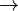# Compounds: further hypotheses

1. Problem and history

Altmann (1989) set up a number of hypotheses on compounds resulting from the synergetic view of language. Up to now, only one of them has been corroborated both theoretically and empirically ($\rightarrow$ Compounds and polysemy). They are presented according to the variable with which compound formation is joined. The problems concerning mathematical modelling, measurement, and testing are here not taken into account; this is preliminarily a mere collect¬ion of hypotheses.

2. Hypotheses

2.1. Hypotheses concerning meaning

Hypothesis 1

The number of compounds in a language (having compounds) decreases proportionally to the measure of semantic correspondence of the components with the compound; or, inversely, the greater the semantic correspondence of the compound with its components, the greater the number of compounds in a language.

Hypothesis 2

The greater the polylexy of a word, the more compounds there are of which it is a component ($\rightarrow$ Compounds and polysemy).

Hypothesis 3

The longer the compound, the greater its semantic correspondence with its components.

Hypothesis 4

The longer a compound, the fewer meanings it has (on average).

2.2. Hypotheses concerning length

Hypothesis 1

The shorter a word, the more frequently it occurs in compounds.

Hypothesis 2

The longer the compound, the shorter its components. This is a simple consequence of Menzerath-Altmann´s law ($\rightarrow$ Hierarchy).

Hypothesis 3

The number of compounds decreases with their increasing length.

2.3. Hypotheses concerning frequency

Hypothesis 1

The more frequent a word, the more compounds it produces.

2.4. Hypotheses concerning cotextuality (polytexty)

Hypothesis 1

The greater the cotextuality of a word, the more compounds it produces.

Hypothesis 2

The longer a compound, the smaller its cotextuality.

2.5. Hypothesis concerning age

Hypothesis 1

The older a word, the more compounds it produces. Here word classes must be treated separately.

3. Derivations

The derivation of individual hypotheses, if it exists, can be found in the pertinent chapter (see the references marked with →).

4. Author: U. Strauss, G. Altmann

5. References

Altmann, G. (1989). Hypotheses about compounds. Glottometrika 10, 100-107.

Altmann, G., Bagheri, D., Goebl, H., Köhler, R., Prün, C. (2002). Einführung in die quantitative Lexikologie. Götingen: Peust & Gutschmidt.